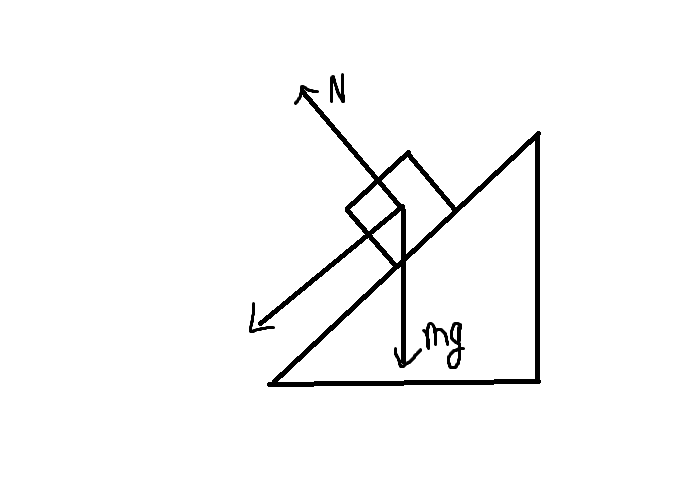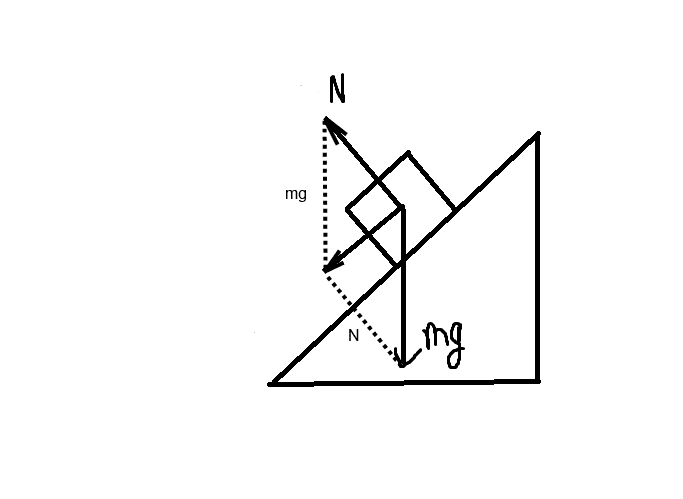# Which of the two is the reason a block slides down a wedge?

• B
• EddiePhys

#### EddiePhysIs the reason the block slides down the plane that the resultant of the normal force N and mg acts along the plane causing it to slide or is it because of the component of mg parallel to the plane acting on the box i.e. mgsin(theta)

Both are the same thing.

Both are the same thing.

Wait, what? How?

Both are the same thing.
Isn't N canceled out by mgcos(x)?

But both N and the component of gravity perpendicular to the slope are perpendicular to the slope.

But both N and the component of gravity perpendicular to the slope are perpendicular to the slope.
But in opposite directions, isn't it?

Pardon me but my mechanics suck

Right. They are in opposite directions and are equal. They add to a net of zero. All that is left after you add normal force plus gravity is the component of gravity parallel to the slope.

The resultant of normal force plus gravity is equal to mg sin theta.

•EddiePhys and Trance-
Right. They are in opposite directions and are equal. They add to a net of zero. All that is left after you add normal force plus gravity is the component of gravity parallel to the slope.

The resultant of normal force plus gravity is equal to mg sin theta.

Oh, I see. Thanks!

Perhaps make your drawing like this...Just to clarify, you do not have 3 forces acting on the block.
You can consider N + mg to be acting, or the resultant to be acting, but not both.

•CWatters